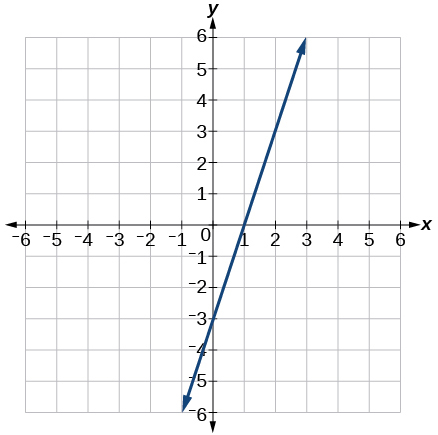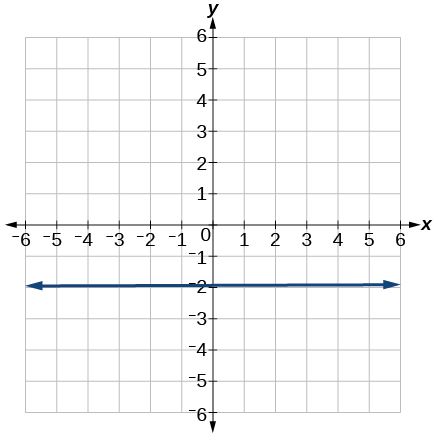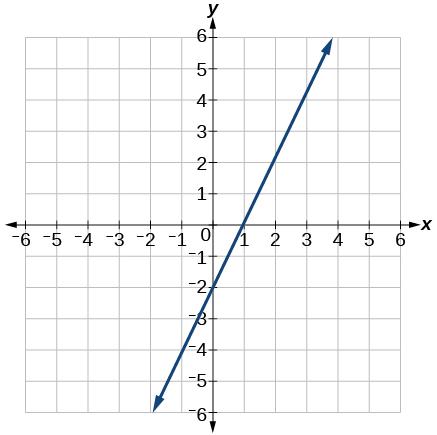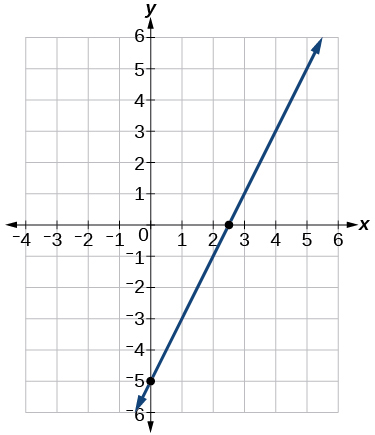# 4.3 Fitting linear models to data  (Page 7/14)

 Page 7 / 14

Determine whether the algebraic equation is linear. $\text{\hspace{0.17em}}6{x}^{2}-y=5$

Determine whether the function is increasing or decreasing.

$f\left(x\right)=7x-2$

Increasing

Determine whether the function is increasing or decreasing.

$g\left(x\right)=-x+2$

Given each set of information, find a linear equation that satisfies the given conditions, if possible.

Passes through $\text{\hspace{0.17em}}\left(\text{7},\text{5}\right)\text{\hspace{0.17em}}$ and $\text{\hspace{0.17em}}\left(\text{3},\text{17}\right)$

$y=-\text{3}x+\text{26}$

Given each set of information, find a linear equation that satisfies the given conditions, if possible.

x -intercept at $\text{\hspace{0.17em}}\left(\text{6},0\right)\text{\hspace{0.17em}}$ and y -intercept at $\text{\hspace{0.17em}}\left(0,\text{1}0\right)$

Find the slope of the line shown in the graph.3

Find the slope of the line graphed.Write an equation in slope-intercept form for the line shown.$y=\text{2}x-\text{2}$

Does the following table represent a linear function? If so, find the linear equation that models the data.

 x –4 0 2 10 g(x) 18 –2 –12 –52

Does the following table represent a linear function? If so, find the linear equation that models the data.

 x 6 8 12 26 g(x) –8 –12 –18 –46

Not linear.

On June 1 st , a company has \$4,000,000 profit. If the company then loses 150,000 dollars per day thereafter in the month of June, what is the company’s profit n th day after June 1 st ?

For the following exercises, determine whether the lines given by the equations below are parallel, perpendicular, or neither parallel nor perpendicular:

$\begin{array}{c}2x-6y=12\\ -x+3y=1\end{array}$

parallel

$\begin{array}{c}y=\frac{1}{3}x-2\\ 3x+y=-9\end{array}$

For the following exercises, find the x - and y - intercepts of the given equation

$7x+9y=-63$

$\left(–9,0\right);\left(0,–7\right)$

$f\left(x\right)=2x-1$

For the following exercises, use the descriptions of the pairs of lines to find the slopes of Line 1 and Line 2. Is each pair of lines parallel, perpendicular, or neither?

Line 1: Passes through $\text{\hspace{0.17em}}\left(5,11\right)\text{\hspace{0.17em}}$ and $\text{\hspace{0.17em}}\left(10,1\right)$

Line 2: Passes through $\text{\hspace{0.17em}}\left(-1,3\right)\text{\hspace{0.17em}}$ and $\text{\hspace{0.17em}}\left(-5,11\right)$

Line 1: $\text{\hspace{0.17em}}m=-2;$ Line 2: $\text{\hspace{0.17em}}m=-2;$ Parallel

Line 1: Passes through $\text{\hspace{0.17em}}\left(8,-10\right)\text{\hspace{0.17em}}$ and $\text{\hspace{0.17em}}\left(0,-26\right)$

Line 2: Passes through $\text{\hspace{0.17em}}\left(2,5\right)\text{\hspace{0.17em}}$ and $\text{\hspace{0.17em}}\left(4,4\right)$

Write an equation for a line perpendicular to $\text{\hspace{0.17em}}f\left(x\right)=5x-1\text{\hspace{0.17em}}$ and passing through the point (5, 20).

$y=-0.2x+21$

Find the equation of a line with a y - intercept of $\text{\hspace{0.17em}}\left(0,2\right)\text{\hspace{0.17em}}$ and slope $\text{\hspace{0.17em}}-\frac{1}{2}.$

Sketch a graph of the linear function $\text{\hspace{0.17em}}f\left(t\right)=2t-5.$Find the point of intersection for the 2 linear functions: $\text{\hspace{0.17em}}\begin{array}{c}x=y+6\\ 2x-y=13\end{array}.$

A car rental company offers two plans for renting a car.

Plan A: 25 dollars per day and 10 cents per mile

Plan B: 50 dollars per day with free unlimited mileage

How many miles would you need to drive for plan B to save you money?

More than 250

## Modeling with Linear Functions

Find the area of a triangle bounded by the y axis, the line $\text{\hspace{0.17em}}f\left(x\right)=10-2x,$ and the line perpendicular to $\text{\hspace{0.17em}}f\text{\hspace{0.17em}}$ that passes through the origin.

A town’s population increases at a constant rate. In 2010 the population was 55,000. By 2012 the population had increased to 76,000. If this trend continues, predict the population in 2016.

118,000

The number of people afflicted with the common cold in the winter months dropped steadily by 50 each year since 2004 until 2010. In 2004, 875 people were inflicted.

Find the linear function that models the number of people afflicted with the common cold C as a function of the year, $\text{\hspace{0.17em}}t.\text{\hspace{0.17em}}$ When will no one be afflicted?

For the following exercises, use the graph in [link] showing the profit, $\text{\hspace{0.17em}}y,$ in thousands of dollars, of a company in a given year, $\text{\hspace{0.17em}}x,$ where $\text{\hspace{0.17em}}x\text{\hspace{0.17em}}$ represents years since 1980.

#### Questions & Answers

write down the polynomial function with root 1/3,2,-3 with solution
if A and B are subspaces of V prove that (A+B)/B=A/(A-B)
write down the value of each of the following in surd form a)cos(-65°) b)sin(-180°)c)tan(225°)d)tan(135°)
Prove that (sinA/1-cosA - 1-cosA/sinA) (cosA/1-sinA - 1-sinA/cosA) = 4
what is the answer to dividing negative index
In a triangle ABC prove that. (b+c)cosA+(c+a)cosB+(a+b)cisC=a+b+c.
give me the waec 2019 questions
the polar co-ordinate of the point (-1, -1)
prove the identites sin x ( 1+ tan x )+ cos x ( 1+ cot x )= sec x + cosec x
tanh`(x-iy) =A+iB, find A and B
B=Ai-itan(hx-hiy)
Rukmini
what is the addition of 101011 with 101010
If those numbers are binary, it's 1010101. If they are base 10, it's 202021.
Jack
extra power 4 minus 5 x cube + 7 x square minus 5 x + 1 equal to zero
the gradient function of a curve is 2x+4 and the curve passes through point (1,4) find the equation of the curve
1+cos²A/cos²A=2cosec²A-1
test for convergence the series 1+x/2+2!/9x3ByByByByByByBy